# Dramatically speed up your R `purrr` functions with the `furrr` package

R’s `purrr` package is an amazing set of tools for applying a function to the items in a vector or list. Although the default for this type of operation in `purrr` is to run each step sequentially the `furrr` package makes it incredibly easy to improve performance by running the steps concurrently (in parallel).

## `purrr` functions run sequentially

In this simple initial example the `map_dbl` function from `purrr` takes the values 1, 2, 3 and 4 and squares them. The computations are done sequentially, one at a time.

``````library(purrr)
map_dbl(1:4, function(x){
x^2
})
##   1  4  9 16``````

But since the individual computations do not depend at all on a previous computation each of these computations could be done at the same time, taking advantage of modern computers’ mutli-core, multi-threaded architecture.

## The `furrr` package can parallelize computations easily

Enter the `furrr` package. With less than a line of code you can convert the sequential process above to a parallel process.

The `furrr` package is a marriage of the `purrr` package and the `future` package which is designed, in part, to provide a simple way to evaluate R expressions asynchronously (as opposed to sequentially).

Thanks for these innovations go to the package creators Davis Vaughan and Matt Dancho (for `furrr`), Henrik Bengtsson (for `future`) and Lionel Henry and Hadley Wickham (for `purrr`).

## Running `purrr` functions in parallel is easy with `furrr`

Here is the same code as before, traditional `purrr` running sequentially.

``````# Sequential
map_dbl(1:4, function(x){
x^2
})
##   1  4  9 16``````

To convert this same set of computations to run in parallel you simply (1) load the `furrr` package, (2) tell R how to set up the parallelization and (3) add `future_` in front of the function name.

``````library(furrr)
future::plan(multiprocess)

# Parallel
future_map_dbl(1:4, function(x){
x^2
})
````##   1  4  9 16````

You can find more detail on `plan()` by looking at the help (`?future::plan()`) but `plan(multiprocess)` will work to parallelize in most instances on both Mac and Windows.

# How do you know it ran in parallel?

Time the computation

If you add a timer you’ll see that `future_map_dbl()` is significantly faster than `map_dbl()`. Note that just squaring a number is fast so I added a 1 second sleep to make the difference clearer. I’m timing it with the `tictoc` package and you can see that the parallel computation is 4x faster.

Note, though, as you’ll see below, in a real-world setting the speed improvements are not always as impressive. It takes time to instantiate a new R process and to copy over the objects needed for the computation. Speed improvements will be greatest in situations where the computations are significantly more time consuming than copying data.

``````library(tictoc)
# Run sequentially

tic() # start timer

map_dbl(1:4, function(x){
Sys.sleep(1)
x^2
})

toc() #end timer
##   1  4  9 16
## 4.022 sec elapsed``````
``````# Run in parallel

tic() # start timer

future_map_dbl(1:4, function(x){
Sys.sleep(1)
x^2
})

toc() # end timer
##   1  4  9 16
## 1.124 sec elapsed``````

Look at activity monitor or task manager

For definitive proof that `future_map_dbl()` runs in multiple R sessions you can look at the Activity Monitor on a Mac or the Task Manager in Windows (on Windows the processes may be listed under the RStudio process). When running `map_dbl()` you will see just one R session listed, meaning just one R instance is running through the computations, each in turn. While running `future_map_dbl()` you’ll see multiple instances of R, in my case I’m seeing four new instances (five total).

Here we only see one instance of rsession, when using `map_dbl()`Here we see five instances of rsession when using `future_map_dbl()`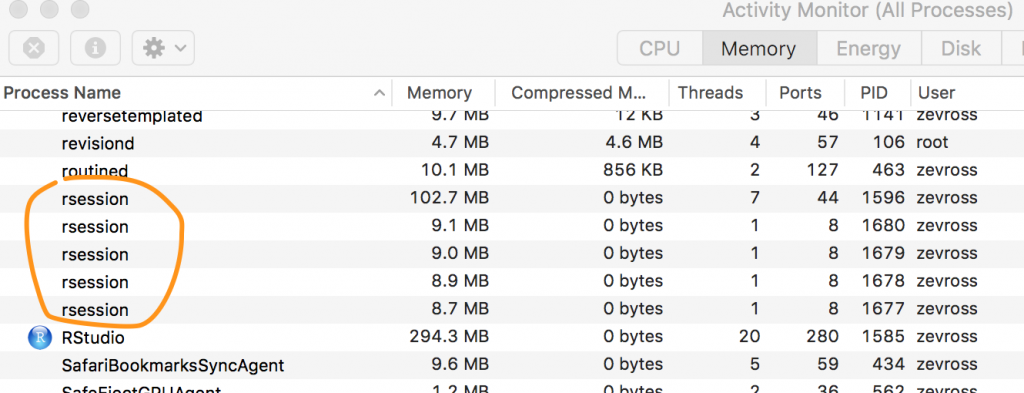## Most `purrr` functions have a parallel equivalent in `furrr`

Nearly all `purrr` functions have been inplemented including `map`, `map2`, `pmap`, `imap`, `walk` as well as more specific versions like `map_dbl`, `map_int` and so on.

All of these will run in parallel

``````future_map_dbl(1:4, ~.^2)
##   1  4  9 16``````
``````l <- list(a = 1:3, b = 4:6, c = 11:13)
future_pmap_dbl(l, function(c, b, a) a / (b + c))
##  0.06666667 0.11764706 0.15789474``````
``````future_imap_chr(sample(10), ~ paste0(.y, ": ", .x))
##   "1: 1"  "2: 5"  "3: 7"  "4: 4"  "5: 2"  "6: 3"  "7: 9"  "8: 10"
##   "9: 6"  "10: 8"``````

## Adding a progress indicator is as easy as typing `.progress = TRUE`

``````future_map_dbl(1:4, function(x){
Sys.sleep(1)
x^2
}, .progress = TRUE)
## Progress: ────────────────────────────── 100%
##   1  4  9 16``````

# A somewhat more real-world example

While this example is still a little contrived, it provides a closer-to-real-world setting for why this might be useful.

In our example we will take a look at state-level and annual trends in births involving twins, triplets etc over a 10-year period 1995-2004.

## Extract the data from Google’s BigQuery

Google’s BigQuery service has a ton of fun open data to work with and, not surprisingly, there is an R package to interact with it. For details you can review the readme. In short, you create an account and a project and then enter your project ID in the query in R. The `"!!YOUR PROJECT ID!!"` in the code should be replaced by the project ID you get from Google.

``````library(bigrquery)
sql <- "SELECT state, year, month,  plurality FROM `publicdata.samples.natality` WHERE year > 1994 and year < 2005"
tb <- bq_project_query("!!YOUR PROJECT ID!!", sql)

You can see that the dataset is reasonably large – about 40 million records representing 40 million births.

``````library(dplyr)
glimpse(births)
## Observations: 39,928,601
## Variables: 4
## \$ state     <chr> "AK", "AK", "AK", "AK", "AK", "AK", "AK", "AK", "AK"...
## \$ year      <int> 1995, 1995, 1995, 1995, 1995, 1995, 1995, 1995, 1995...
## \$ month     <int> 1, 1, 1, 1, 1, 1, 1, 1, 1, 1, 1, 1, 1, 1, 1, 1, 1, 1...
## \$ plurality <int> 1, 1, 1, 1, 1, 1, 1, 1, 1, 1, 1, 1, 1, 1, 1, 1, 1, 1...``````

The field `plurality` refers to the number of babys, here are the counts.

``````group_by(births, year, plurality) %>%
summarise(counts = n()) %>%
## # A tibble: 5 x 11
##   plurality `1995` `1996` `1997` `1998` `1999` `2000` `2001` `2002` `2003`
##       <int>  <int>  <int>  <int>  <int>  <int>  <int>  <int>  <int>  <int>
## 1         1 3.80e6 3.79e6 3.77e6 3.83e6 3.84e6 3.94e6 3.90e6 3.89e6 3.96e6
## 2         2 9.68e4 1.01e5 1.04e5 1.11e5 1.14e5 1.19e5 1.21e5 1.25e5 1.29e5
## 3         3 4.57e3 5.30e3 6.16e3 6.92e3 6.75e3 6.74e3 6.90e3 6.91e3 7.12e3
## 4         4 3.65e2 5.64e2 5.10e2 6.27e2 5.12e2 5.06e2 5.05e2 4.34e2 4.68e2
## 5         5 5.70e1 8.10e1 7.90e1 7.90e1 6.70e1 7.70e1 8.50e1 6.90e1 8.50e1
## # ... with 1 more variable: `2004` <int>``````

## Split the data by year

Here is the contrived portion. You could do the upcoming calculations on the full dataset without breaking it up by year and you could even do these calculations in your SQL call to BigQuery. But what we will do instead is split up our data set into a list based on year – each element in the list is a data frame for one year’s worth of data.

``````births_split <- split(births, births\$year)
length(births_split) # one element per year
##  10``````
``````# look at the data for one year
## # A tibble: 6 x 4
##   state  year month plurality
##   <chr> <int> <int>     <int>
## 1 AK     1995     1         1
## 2 AK     1995     1         1
## 3 AK     1995     1         1
## 4 AK     1995     1         1
## 5 AK     1995     1         1
## 6 AK     1995     1         1``````

## Compute the proportion of multiple births and compare `purrr` and `furrr`

We will compute a table like the one below – we need a count of births involving multiple babies and a total count of births. The number we’re interested in computing is `multiple_births_proportion` which is the proportion of all births that are multiple births.

``````## # A tibble: 612 x 6
##    state  year month multiple_births_co… total_births multiple_births_pro…
##    <chr> <int> <int>               <int>        <int>                <dbl>
##  1 AK     1995     1                  16          795                0.02
##  2 AK     1995     2                  23          736                0.031
##  3 AK     1995     3                  31          895                0.035
##  4 AK     1995     4                  20          840                0.024
##  5 AK     1995     5                  26          901                0.029
##  6 AK     1995     6                  24          908                0.026
##  7 AK     1995     7                  20          852                0.023
##  8 AK     1995     8                  23          899                0.026
##  9 AK     1995     9                  23          893                0.026
## 10 AK     1995    10                  16          829                0.019
## # ... with 602 more rows``````

We will use `map_dfr` (and the `furrr` companion `future_map_dfr`) to cycle through the data frames in the list (remember each data frame is one year of births). The `_dfr` tells the functions to return a single data frame – similar to `rbind()` or `bind_rows()`.

We could use the `tictoc` package to time again but there are variations in computer processing time so a single run might not give a good portrait. Instead we will time with the `microbenchmark`’s `microbenchmark` function which allows us to run multiple iterations of the same code and provides an average of the time the computations take.

Run sequentially (the default)

``````time_serial <- microbenchmark::microbenchmark({

birth_results_serial <- purrr::map_dfr(births_split, function(.data){

.data %>%
mutate(morethan1 = plurality > 1) %>%
group_by(state, year, month, morethan1) %>%
summarize(multiple_births_count = n()) %>%
mutate(total_births = sum(multiple_births_count),
multiple_births_proportion =
round(multiple_births_count/total_births, 3)) %>%
arrange(state, year, month, morethan1)  %>%
filter(morethan1)

})

}, times = 10) # times = 10 tells it run the code 10 times
summary(time_serial)\$median # in seconds here
##  2.442766``````

Run in parallel (using `furrr`)

``````plan(multiprocess)
time_parallel <- microbenchmark::microbenchmark({

birth_results_parallel <- furrr::future_map_dfr(births_split, function(.data){

.data %>%
mutate(morethan1 = plurality > 1) %>%
group_by(state, year, month, morethan1) %>%
summarize(multiple_births_count = n()) %>%
mutate(total_births = sum(multiple_births_count),
multiple_births_proportion =
round(multiple_births_count/total_births, 3)) %>%
ungroup() %>%
arrange(state, year, month, morethan1)  %>%
filter(morethan1)

})

}, times = 10)

summary(time_parallel)\$median # in seconds here
##  2.057291``````

Confirm the two resulting tables are the same

``````identical(birth_results_serial, birth_results_parallel)
##  TRUE``````

Confirm table looks correct

``head(birth_results_parallel)``
``````## # A tibble: 6 x 7
##   state  year month morethan1 multiple_births… total_births
##   <chr> <int> <int> <lgl>                <int>        <int>
## 1 AK     1995     1 TRUE                    16          795
## 2 AK     1995     2 TRUE                    23          736
## 3 AK     1995     3 TRUE                    31          895
## 4 AK     1995     4 TRUE                    20          840
## 5 AK     1995     5 TRUE                    26          901
## 6 AK     1995     6 TRUE                    24          908
## # ... with 1 more variable: multiple_births_proportion <dbl>``````

Time difference

In this particular example, the time saved is less impressive, most likely due to the fact that the computation time for this example is small relative to the data size. It takes time for R and your computer to open new R sessions and to copy the data over. But we still see a time savings of approximately 19%.

``````# Time serial takes 1.187 (19%) more time to run
summary(time_serial)\$median/summary(time_parallel)\$median``````
``##  1.18737``

A couple of notes about Windows machines

We found that there were two issues for Windows users in our office. First, one person got an error related to the data exceeding the maximum allowed size. To fix this issue she used:

``options(future.globals.maxSize= 891289600)``

We also noticed on Windows machines the `dplyr` code ran slower (this was not true on Macs). We suspect this was due to slower speed setting up the new threads and copying the data given the the small bit of code at the beginning of this post ran quicker in parallel and that the `future_map_dfr` code ran more slowly even if we commented out all of the actual processing code (i.e., left the section within `future_map_dfr` empty).

What does the multiple births data look like?

In case you’re curious about the patterns in multiple births, I’ve provided a few visualizations of the resulting data.

``birth_results <- birth_results_parallel``

## Proportion of multiple births overall

There is significant spread in the proportion of births resulting in multiple babies with an average of 0.03.

``````library(ggplot2)
birth_results %>%
ggplot(aes(multiple_births_proportion))+
geom_histogram(color = "white") +
geom_vline(xintercept = median(birth_results\$multiple_births_proportion), color = "forestgreen", size = 1.5) +
ggtitle("Proportion of births with more than one baby (by state, year and month)")``````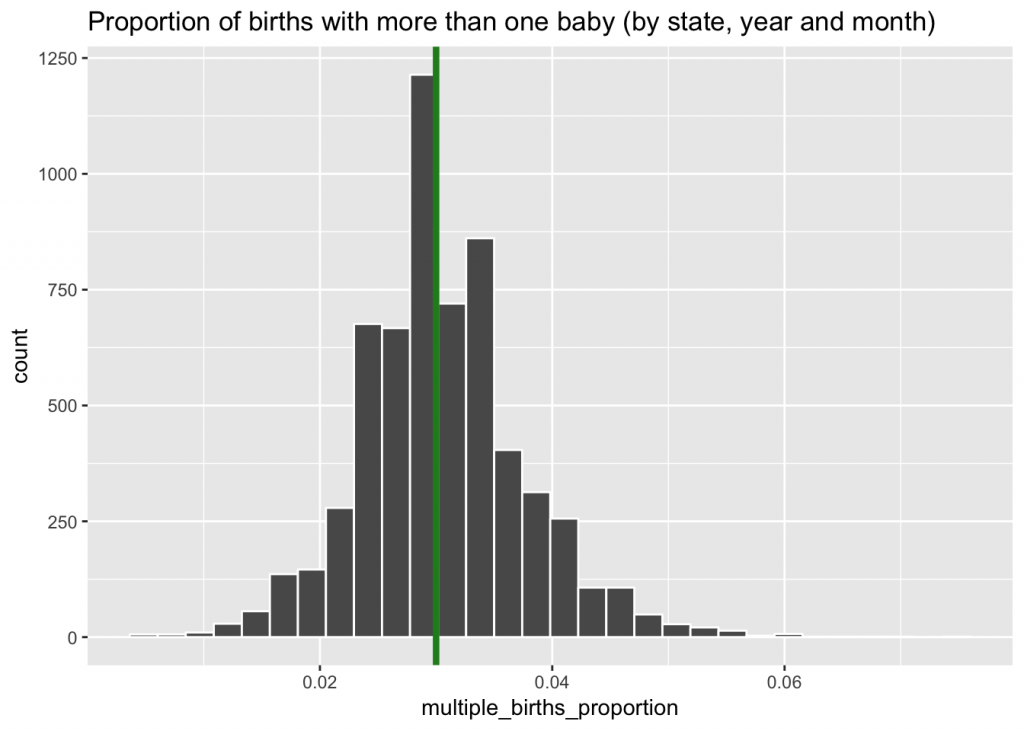## More detail: proportion of multiple births by state, year and season

For reference we will compute (and add to the plots) the state-level medians (across all months and years). We will also do a rough assignment of month to season.

The `forcats` package is useful for working with categorical data, in this case we’re reordering the state factor levels by average proportion of multiple births (`avg`) with `fct_reorder()` so that the states will be organized in the plots and then we’re collapsing months into season with `fct_collapse()`.

``````birth_results <- birth_results %>%
group_by(state) %>%
mutate(avg = median(multiple_births_proportion),
month = as.character(month)) %>%
ungroup() %>%
mutate(state = forcats::fct_reorder(state, avg)) %>%
mutate(season = forcats::fct_collapse(month,
winter = c("1", "2", "12"),
spring = c("3", "4","5"),
summer = c("6", "7", "8"),
fall = c("9", "10", "11")
))``````

There is a clear difference in states. There is also a hint of a yearly difference (see how the weight of the points is to the left of the black medians in 1995 and to the right in 2004) but I can’t see a seasonal difference.

``````birth_results %>%
ggplot(aes(state, multiple_births_proportion)) +
geom_point(aes(color = season)) +
coord_flip()+
geom_point(data = distinct(birth_results, state, avg), aes(state, avg)) +
facet_wrap(~year) +
theme(axis.text.y = element_text(size = 6))``````

Click on this image to see a bigger version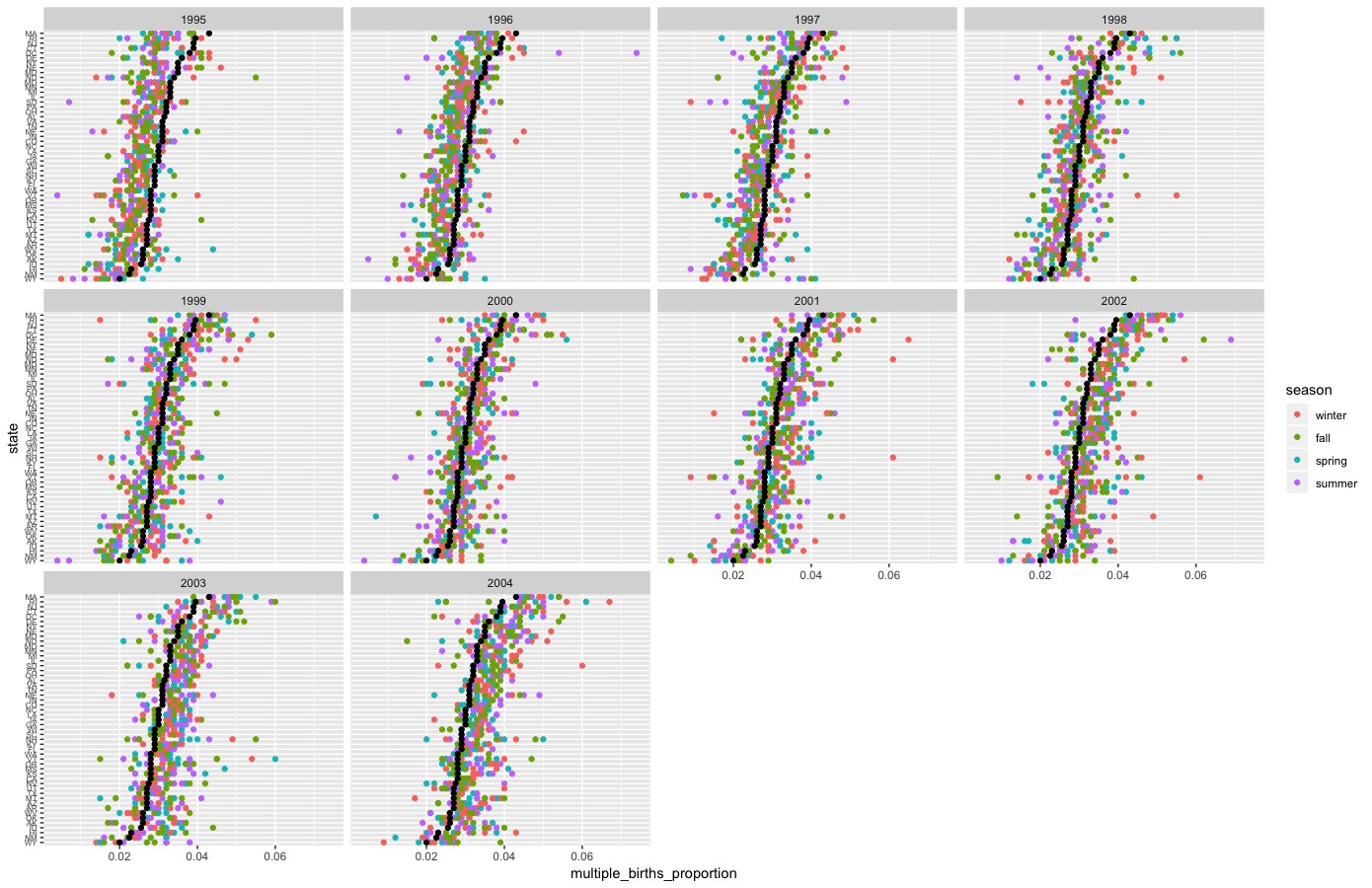Significant state-level differences

We can see that Massachusetts, Rhode Island, New Jersey and Connecticut have nearly double the rate of multiple births than Wyoming, New Mexico and Hawaii.

``````state_medians <- birth_results %>%
group_by(state) %>%
summarize(avg = median(multiple_births_proportion)) %>%
arrange(avg)``````
``````head(state_medians)
## # A tibble: 6 x 2
##   state    avg
##   <fct>  <dbl>
## 1 WY    0.02
## 2 NM    0.0225
## 3 HI    0.023
## 4 ID    0.0255
## 5 AK    0.026
## 6 OK    0.026``````
``````tail(state_medians)
## # A tibble: 6 x 2
##   state    avg
##   <fct>  <dbl>
## 1 DE    0.036
## 2 DC    0.038
## 3 CT    0.039
## 4 NJ    0.039
## 5 RI    0.0395
## 6 MA    0.043``````

## Closer inspection of yearly and seasonal differences

We can tease out yearly and seasonal differences with box plots organized in a couple of different ways. There seems to be a pretty clear yearly difference but I’m still not seeing a consistent seasonal difference (though in another setting I’d probably take a closer look).

``````birth_results %>%
ggplot(aes(season, multiple_births_proportion))+
geom_boxplot(aes(color = factor(year)))``````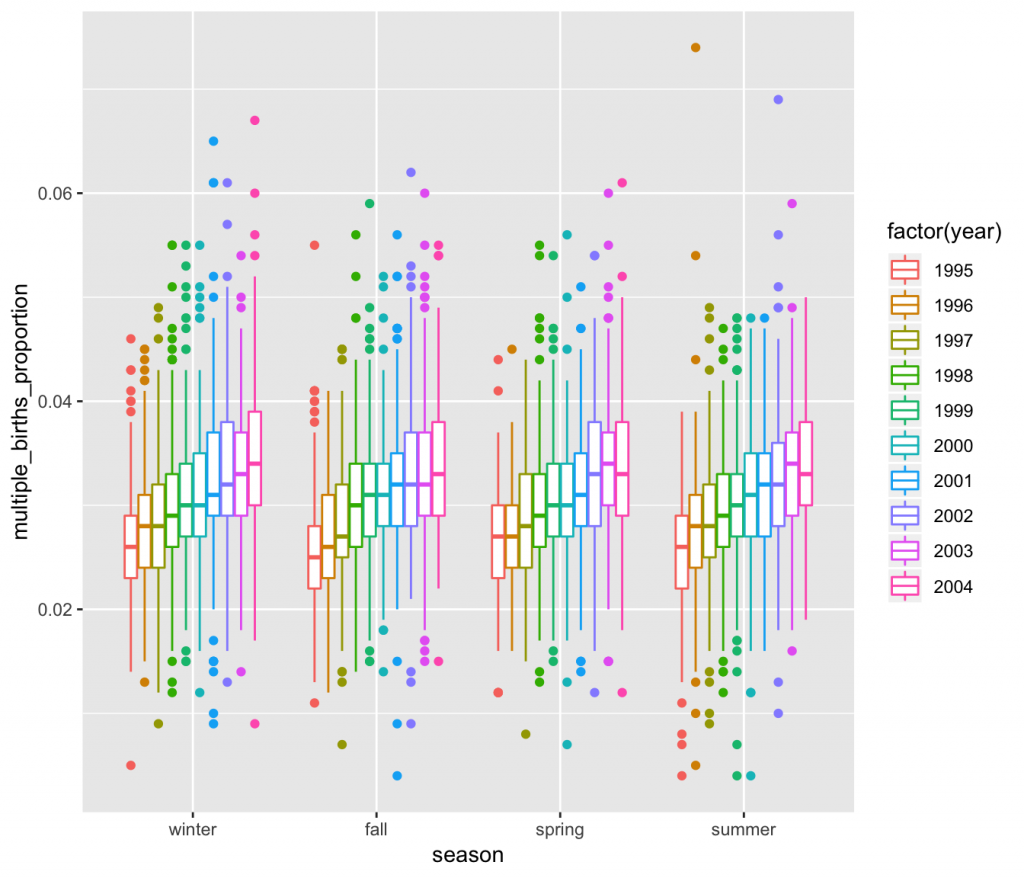``````birth_results %>%
ggplot(aes(factor(year), multiple_births_proportion))+
geom_boxplot(aes(color = factor(season)))``````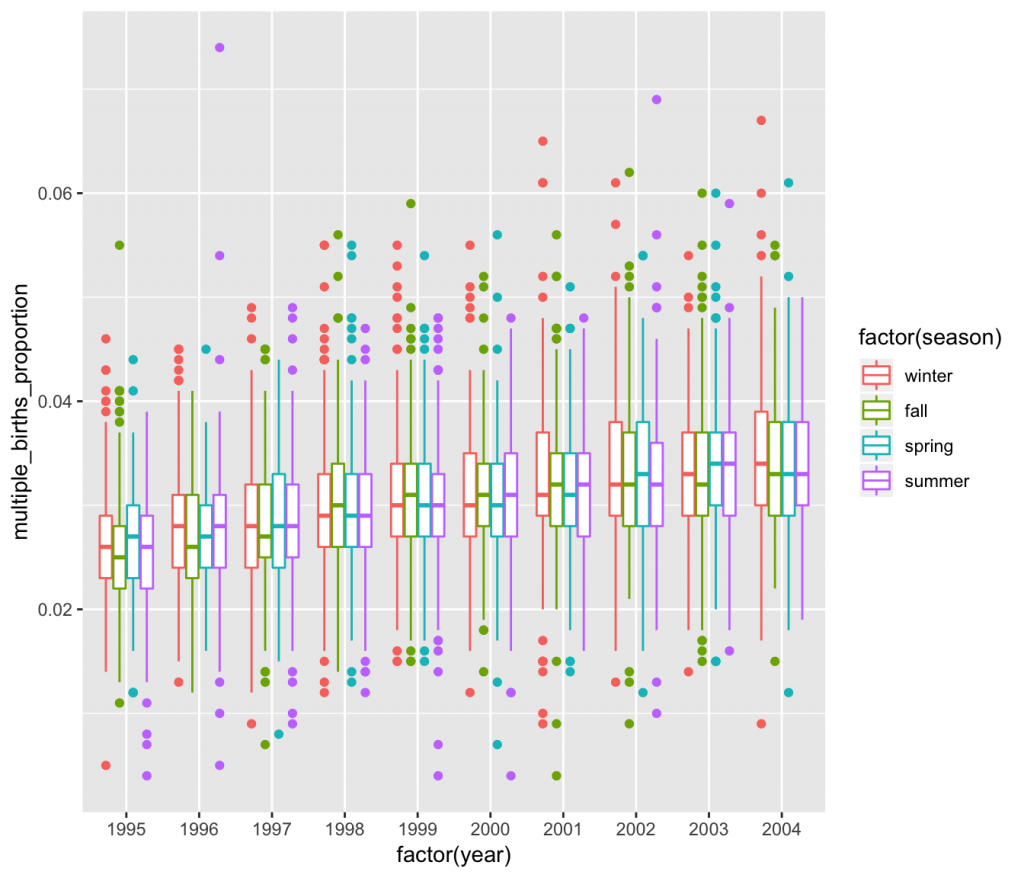Finally we can take a look at the yearly trend. We definitely see a clear (probably linear) upward trend in the proportion of multiple births. This is consistent with findings elsewhere may be due to an increasing average age at which women have children or increasing use of fertility drugs.

``````birth_results %>%
ggplot(aes(year, multiple_births_proportion))+
geom_jitter(width = 0.2, alpha = 0.1) +
stat_smooth(method = "lm")``````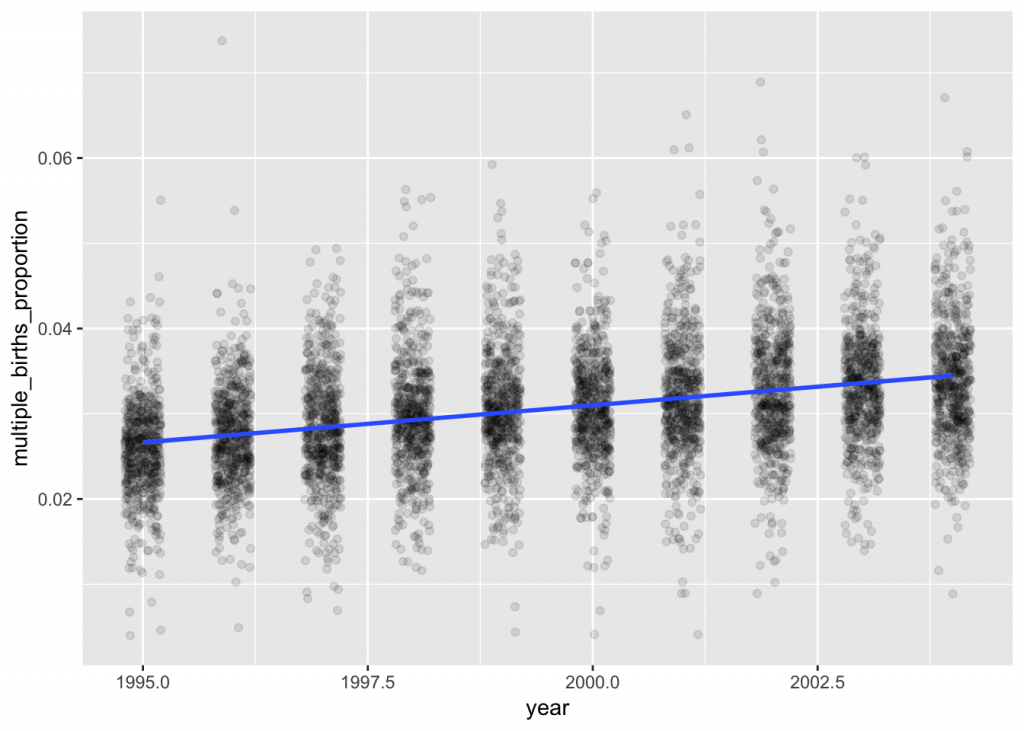# Conclusion

With less than a line of code you can convert your sequential `purrr` code into code that runs in parallel using the `furrr` package. Running the code can have significant performance benefits though the benefits you see will depend on the amount of computation and the amount of data you’re working with. Nearly all `purrr` functions have a corresponding `furrr` equivalent so pretty much anything you run with `purrr` can be parallelized.